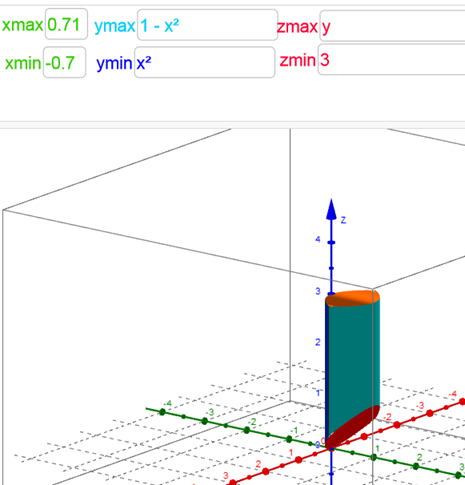# Determine the region that lies between two planes and two cylinder

Matea Pjanic shared this question 3 years ago

In 3D I need to determine the region bounded by two planes z=3 and z=y and two cylinders, y=x^2 and y=1-x^2 ?

There are four conics that are in the intersection (black in the picture) :

X=(0,1,3)+(-0.5t-0.25t^2,0)

X=(0,1,1)+(-0.35t,-0.13t^2,-0.13t^2)

X=(0,0,0)+(-0.35t,0.13t^2,0.13t^2)

X=(0,0,3)+(-0.5t,0.25t^2,0)

but is there a way to find the region that is bounded by those conics?2https://www.geogebra.org/m/...1

Thank you mathmagic, that is exactly what I need, but I need the ggb file and for some reason downloading Applet1.ggb file doesnt work, neither I can open it in app. Maybe you can tell what is the problem?11

It works! Thanks a lot